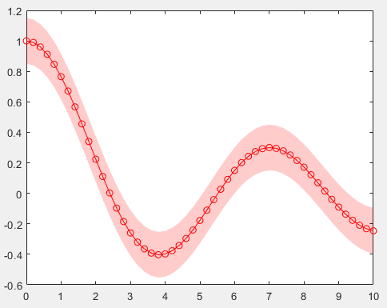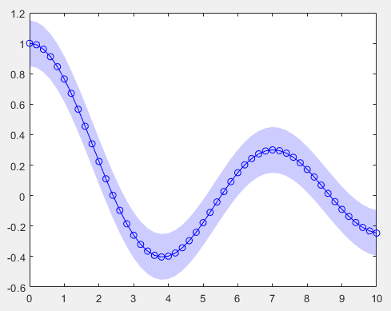## 图开发概述

• 为用户提供方便的接口 - 当用户要自定义图的某个方面时，他们可以设置属性，而不必修改和重新运行图形代码。用户可以在命令行修改属性或在属性检查器中检查它们。

• 封装算法和基本图形对象 - 实现用于执行计算和管理基础图形对象的方法。以这种方式组织您的代码，您可以对用户隐藏实现细节。

### 图类的结构

`classdef ConfidenceChart < matlab.graphics.chartcontainer.ChartContainer`

（推荐）

（推荐）

• `Access = private`

• `Transient`

• `NonCopyable`

### 隐式构造函数方法

```c = ConfidenceChart(gcf,'XData',[1 2 3],'YData',[4 5 6]) c = ConfidenceChart('XData',[1 2 3],'YData',[4 5 6])```

### 公共和私有属性块

• 用于存储面向用户界面的组件的公共属性块

• 用于存储要隐藏的实现细节的私有属性块

```properties XData = NaN YData = NaN ConfidenceMargin = 0.15 MarkerSymbol = 'o' Color = [1 0 0] end properties(Access = private,Transient,NonCopyable) LineObject PatchObject end ```

### setup 方法

• 调用绘图函数来创建要在图中使用的基本图形对象。

• 将绘图函数返回的基本对象作为私有属性存储在图对象上。

• 配置基本图形对象。

• 配置坐标区。

`setup` 方法中调用绘图函数时，请为坐标数据指定临时值（例如 `NaN`）。另外，还需要为与类的公共属性相对应的其他参数指定临时值。这样做可以避免在 `setup``update` 方法中设置相同的属性值。

• 两个公共属性，分别名为 `XData``YData`，用于存储线的 x 和 y 坐标

• 两个私有属性，分别名为 `LineObject``PatchObject`

`setup` 方法通过调用 `getAxes` 方法获取坐标区对象。然后，它会调用 `patch` 函数，并将输出存储在 `PatchObject` 属性中。在调用 `plot` 函数创建 `LineObject` 属性之前，下一行代码将坐标区的保留状态设置为 `'on'`。最后一行代码将坐标区 hold 状态设置回 `'off'`

```function setup(obj) % Get the axes ax = getAxes(obj); % Create Patch and Line objects obj.PatchObject = patch(ax,NaN,NaN,'r','FaceAlpha',0.2,... 'EdgeColor','none'); hold(ax,'on') obj.LineObject = plot(ax,NaN,NaN); % Turn hold state off hold(ax,'off') end```

### update 方法

• 两个公共属性，分别名为 `XData``Color`

• 两个名为 `LineObject``PatchObject` 的私有属性

`update` 方法会更新 `Line``Patch` 对象的 `XData``Color` 属性。

```function update(obj) % Update XData of line object obj.LineObject.XData = obj.XData; % Update patch XData x = obj.XData; obj.PatchObject.XData = [x x(end:-1:1)]; % Update line object colors obj.LineObject.Color = obj.Color; obj.PatchObject.FaceColor = obj.Color; end```

### 示例：置信边界图

`ChartContainer` 基类派生而来。

`classdef ConfidenceChart < matlab.graphics.chartcontainer.ChartContainer`

``` properties XData = NaN YData = NaN ConfidenceMargin = 0.15 MarkerSymbol = 'o' Color = [1 0 0] end```

``` properties(Access = private,Transient,NonCopyable) LineObject PatchObject end```

``` methods(Access = protected) function setup(obj) % get the axes ax = getAxes(obj); % Create Patch and Line objects obj.PatchObject = patch(ax,NaN,NaN,'r','FaceAlpha',0.2,... 'EdgeColor','none'); hold(ax,'on') obj.LineObject = plot(ax,NaN,NaN); % Turn hold state off hold(ax,'off') end```

``` function update(obj) % Update XData and YData of Line obj.LineObject.XData = obj.XData; obj.LineObject.YData = obj.YData; % Update patch XData and YData x = obj.XData; obj.PatchObject.XData = [x x(end:-1:1)]; y = obj.YData; c = obj.ConfidenceMargin; obj.PatchObject.YData = [y+c y(end:-1:1)-c]; % Update colors obj.LineObject.Color = obj.Color; obj.PatchObject.FaceColor = obj.Color; % Update markers obj.LineObject.Marker = obj.MarkerSymbol; end end end```

```x = 0:0.2:10; y = besselj(0,x); c = ConfidenceChart('XData',x,'YData',y,'ConfidenceMargin',0.15);````c.Color = [0 0 1];`### 支持常用图形功能

`matlab.graphics.chartcontainer.mixin.Legend`

`matlab.graphics.chartcontainer.mixin.Colorbar`